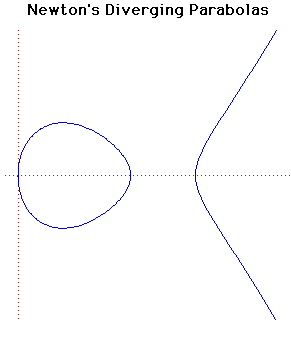# Curves

### Newton's Diverging ParabolasCartesian equation:
$ay^{2} = x(x^{2} - 2bx + c), a > 0$

### Description

Newton's classification of cubic curves appears in Curves by Sir Isaac Newton in Lexicon Technicum by John Harris published in London in 1710. In this classification of cubics, Newton gives four classes of equation. The third class of equations is the one given above which Newton divides into five species. Of this third case Newton says:

In the third Case the Equation was $yy = ax^{3} + bxx + cx + d$ and defines a Parabola whose Legs diverge from one another, and run out infinitely contrary ways.

The case divides into five species and Newton gives a typical graph for each species. The five types depend on the roots of the cubic in $x$ on the right hand side of the equation.

(i) All the roots are real and unequal : then the Figure is a diverging Parabola of the Form of a Bell, with an Oval at its vertex .
This is the case for the graph drawn above.

(ii) Two of the roots are equal : a Parabola will be formed, either Nodated by touching an Oval, or Punctate, by having the Oval infinitely small .

(iii) The three roots are equal : this is the Neilian Parabola, commonly called Semi-cubical .

(iv) Only one real root : If two of the roots are impossible, there will be a Pure Parabola of a Bell-like Form .

### Associated Curves

Definitions of the Associated curves